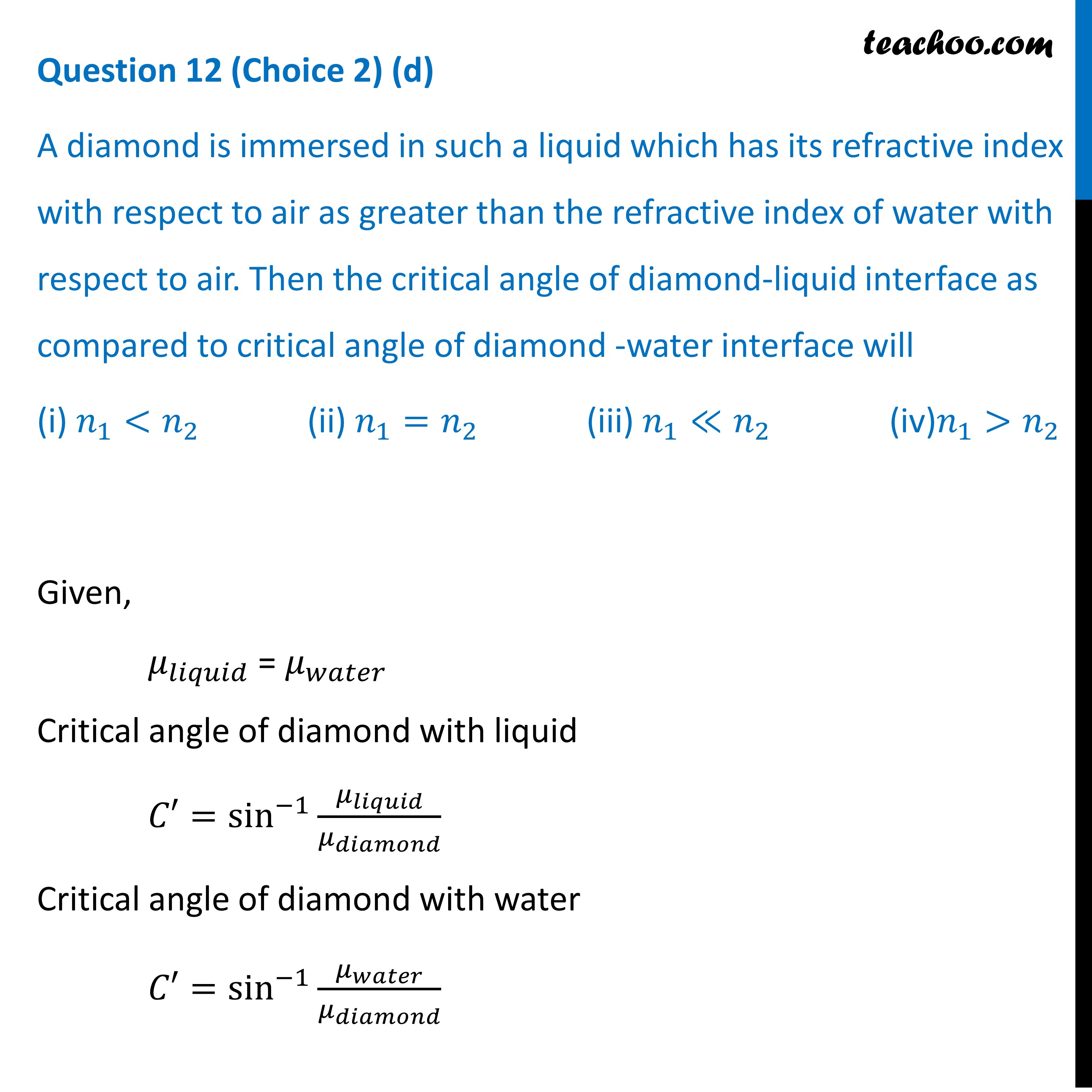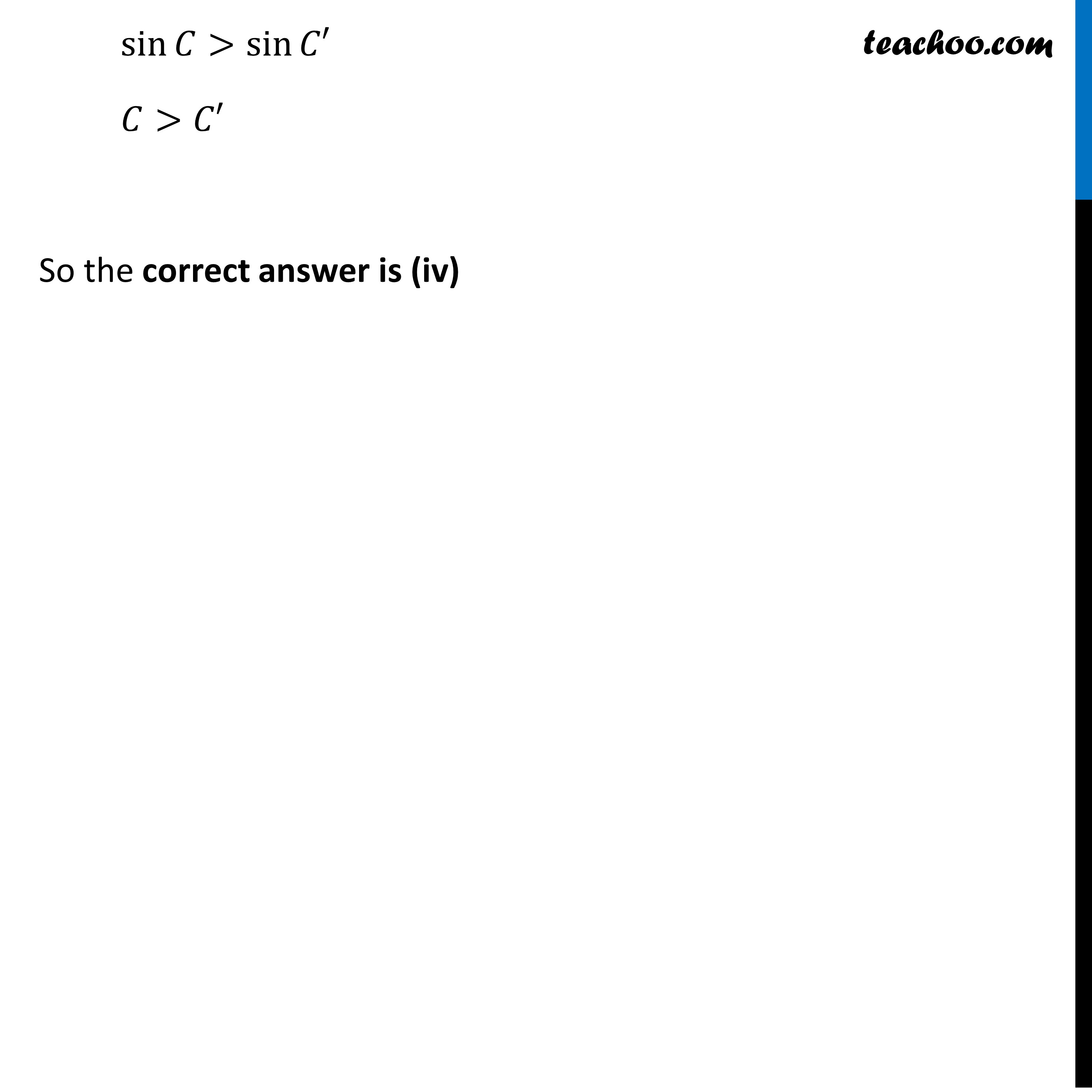CBSE Class 12 Sample Paper for 2022 Boards [Term 2] - Physics

Physics Class 12
Solutions to CBSE Sample Paper - Physics Class 12

## (iv) increase.Learn in your speed, with individual attention - Teachoo Maths 1-on-1 Class

### Transcript

Question 12 (Choice 2) (d) A diamond is immersed in such a liquid which has its refractive index with respect to air as greater than the refractive index of water with respect to air. Then the critical angle of diamond-liquid interface as compared to critical angle of diamond -water interface will (i) 𝑛_1<𝑛_2 (ii) 𝑛_1=𝑛_2 (iii) 𝑛_1≪𝑛_2 (iv)𝑛_1>𝑛_2 Given, 𝜇_𝑙𝑖𝑞𝑢𝑖𝑑 = 𝜇_𝑤𝑎𝑡𝑒𝑟 Critical angle of diamond with liquid 𝐶′=sin^(−1)⁡〖𝜇_𝑙𝑖𝑞𝑢𝑖𝑑/𝜇_𝑑𝑖𝑎𝑚𝑜𝑛𝑑 〗 Critical angle of diamond with water 𝐶′=sin^(−1)⁡〖𝜇_𝑤𝑎𝑡𝑒𝑟/𝜇_𝑑𝑖𝑎𝑚𝑜𝑛𝑑 〗 sin⁡𝐶>sin⁡𝐶′ 𝐶>𝐶′ So the correct answer is (iv)